# LED panel

We made an LED panel that reacts to temperature, force, and sound.

BeginnerFull instructions provided6 hours105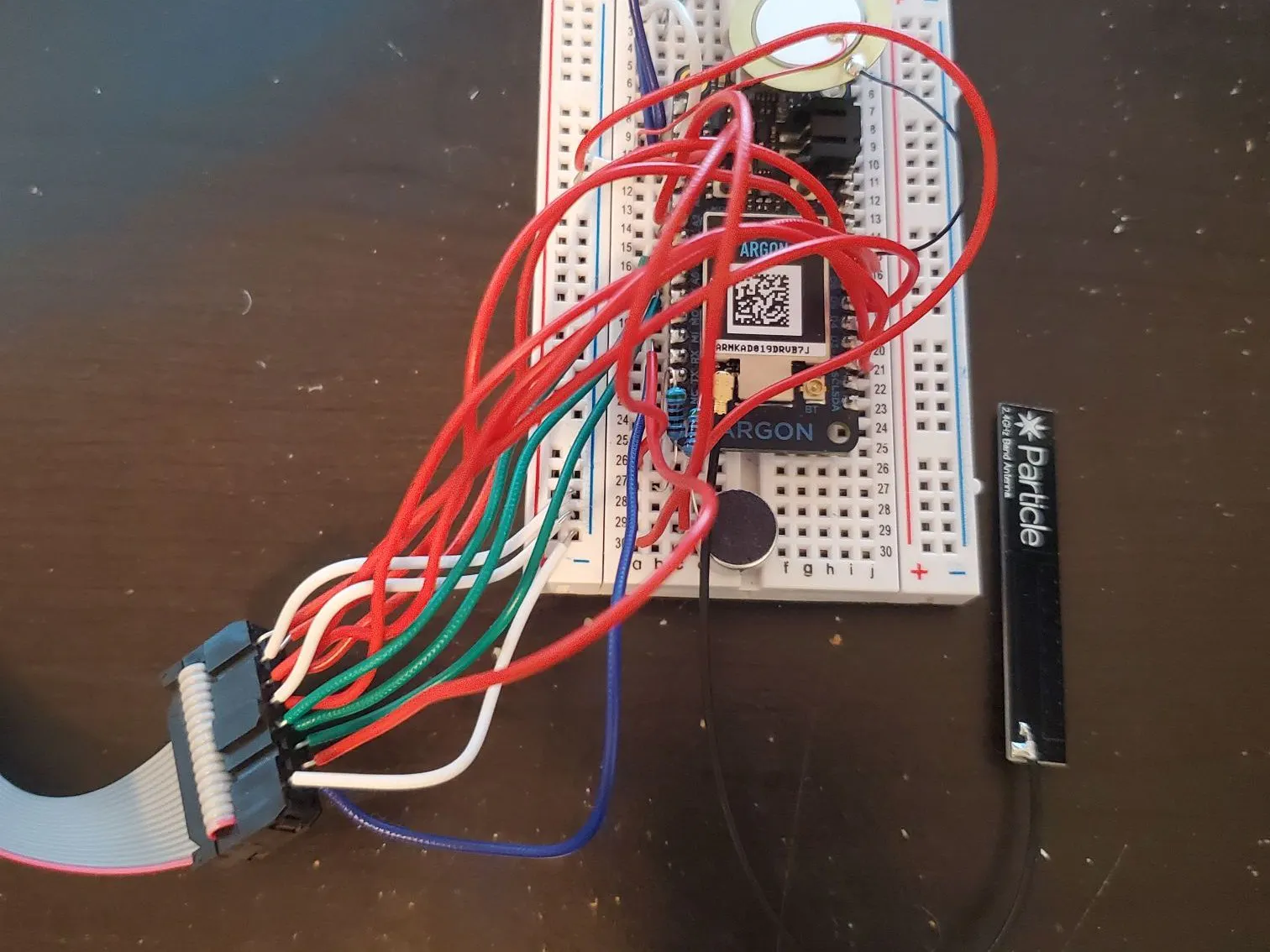## Things used in this project

### Hardware components

 Adafruit 32x32 RGB LED Matrix Panel
×1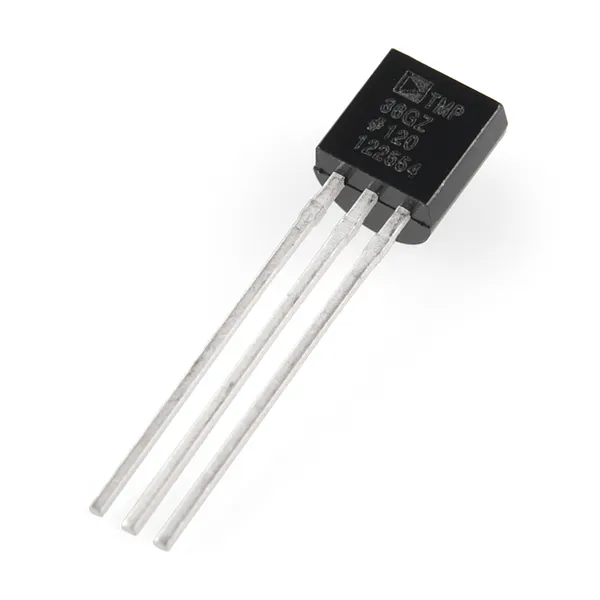Temperature Sensor
×1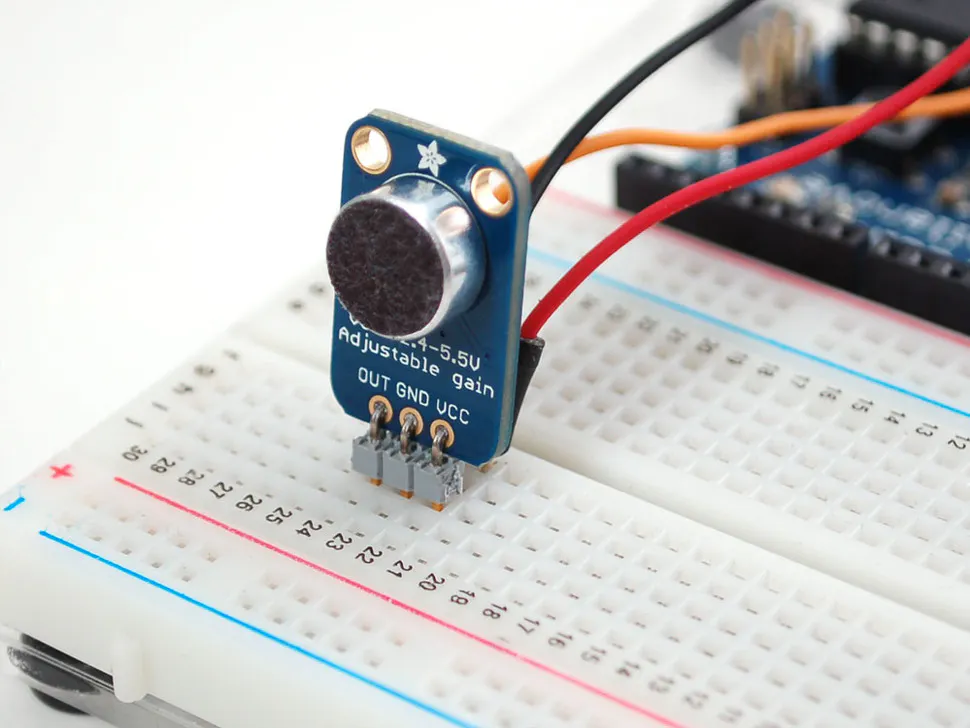×1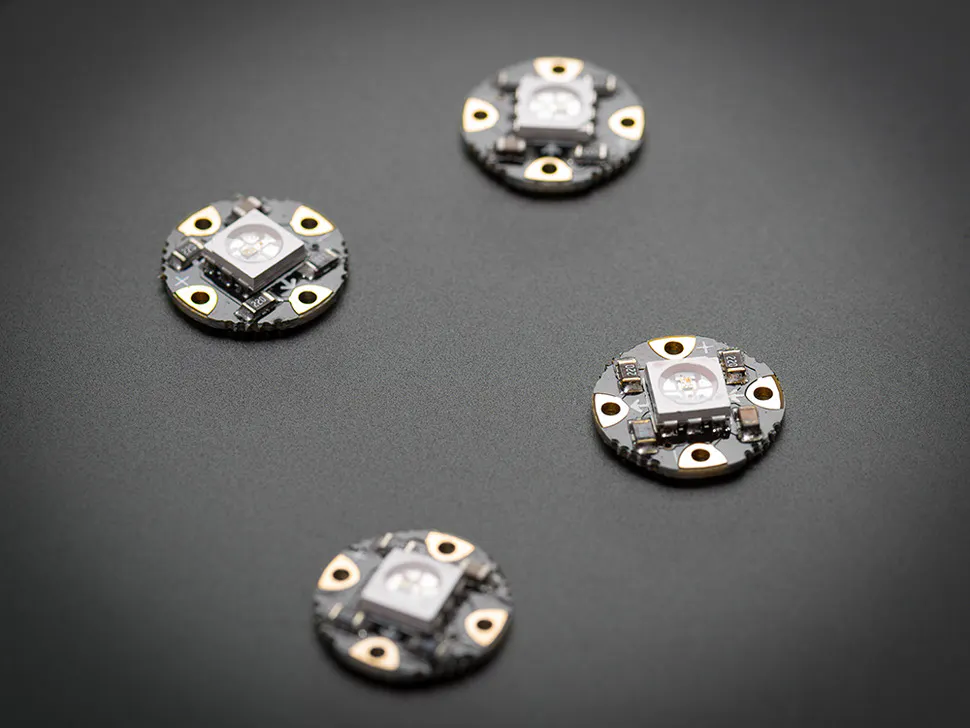Adafruit Flora RGB Neopixel LEDs- Pack of 4
×1

### Software apps and online servicesParticle Build Web IDE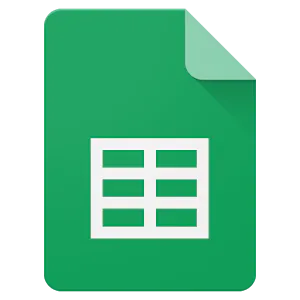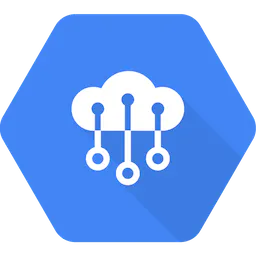## Schematics

### IOT circuit schematic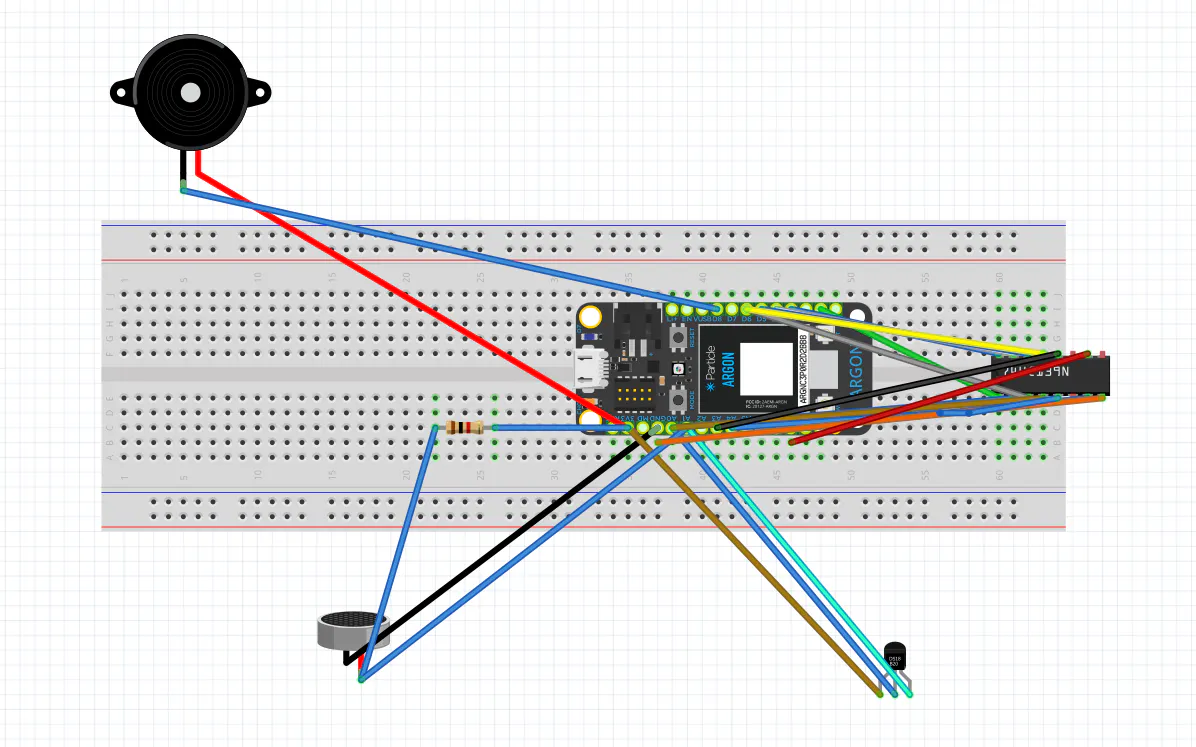## Code

### 3sensorRGBLEDs

C/C++
Code defines sensor and LED pins and then activates LED based on data from sensors. Sensor data is uploaded and webhooked to log and chart data.
```// This #include statement was automatically added by the Particle IDE.

#define DHTPIN A0
#define DHTTYPE DHT11
#define SNDPIN A1
#define FRCPIN A2
#define R1PIN D3
#define B1PIN D4
#define R2PIN D5
#define B2PIN D6
#define UPDATE 500ms

//Change this to the max expected value of sound input
float soundMax = 4096;
//Change this to the max expected value of force input
float forceMax = 4096;

DHT dht(DHTPIN, DHT11, 8);

void setup() {
Particle.publish("Testing logging");

dht.begin();

pinMode(SNDPIN, INPUT);

pinMode(FRCPIN, INPUT);

pinMode(R1PIN, OUTPUT);
pinMode(B1PIN, OUTPUT);
pinMode(R2PIN, OUTPUT);
pinMode(B2PIN, OUTPUT);
}

void loop() {
int temp = dht.getTempCelcius();     // read temperature

Particle.publish("Temperature", String(temp));

Particle.publish("Sound", String(sound));

Particle.publish("Force", String(force));

String out = String::format("{\"temperature\":%d,\"sound\":%d,\"force\":%d}",temp, sound, force);

Particle.publish("blob", out , PRIVATE, WITH_ACK);

float soundFactor = sound/soundMax;
float forceFactor = force/forceMax;

setRB(soundFactor * (temp*10), soundFactor * (255 - (temp*10)), R1PIN, B1PIN);

delay(UPDATE);

setRB(forceFactor * (temp*10), forceFactor * (255 - (temp*10)), R2PIN, B2PIN);

delay(UPDATE);
}

void setRB(int red, int blue, int pinR, int pinB) {
if(red < 0) {
red = 0;
}
if(red > 255) {
red = 255;
}
if(blue < 0) {
blue = 0;
}
if(blue > 255) {
blue = 255;
}
analogWrite(pinR, red);
analogWrite(pinB, blue);
}
```

JavaScript
```function doPost(e) {

//Return if null
if( e == undefined ) {
Logger.log("no data");
return HtmlService.createHtmlOutput("need data");
}

//Parse the JSON data
var event = JSON.parse(e.postData.contents);
var data = JSON.parse(event.data);

//Get the last row without data
var lastRow = Math.max(sheet.getLastRow(),1);
sheet.insertRowAfter(lastRow);

//Get current timestamp
var timestamp = new Date();

//Insert the data into the sheet
sheet.getRange(lastRow + 1, 1).setValue(event.published_at);
sheet.getRange(lastRow + 1, 2).setValue(data.temperature);
sheet.getRange(lastRow + 1, 3).setValue(data.sound);
sheet.getRange(lastRow + 1, 4).setValue(data.force);

}
```

## Credits

### Alyson Witte

1 project • 3 followers

### Jordon Waters

1 project • 3 followers
Thanks to Yazeed Hawari.